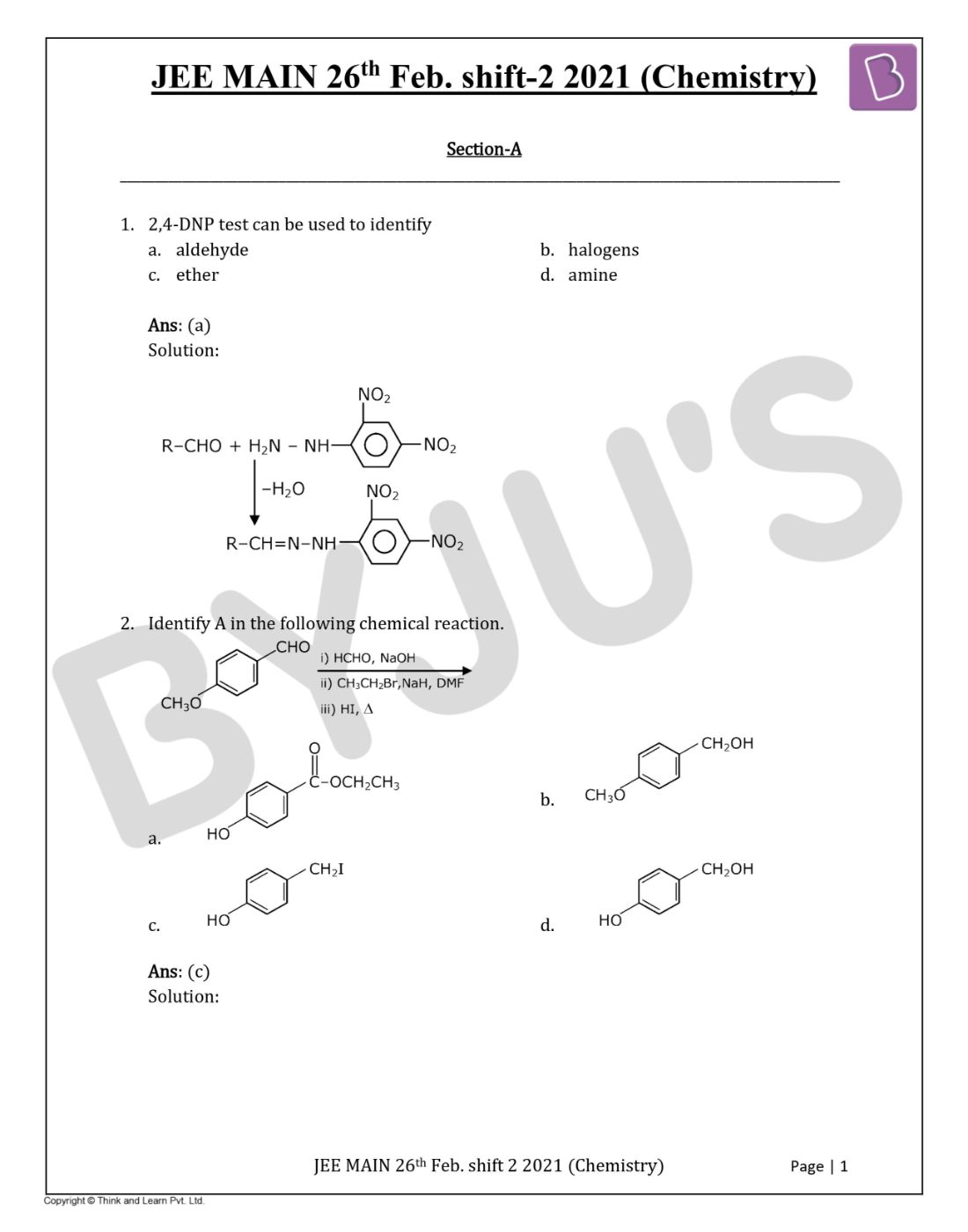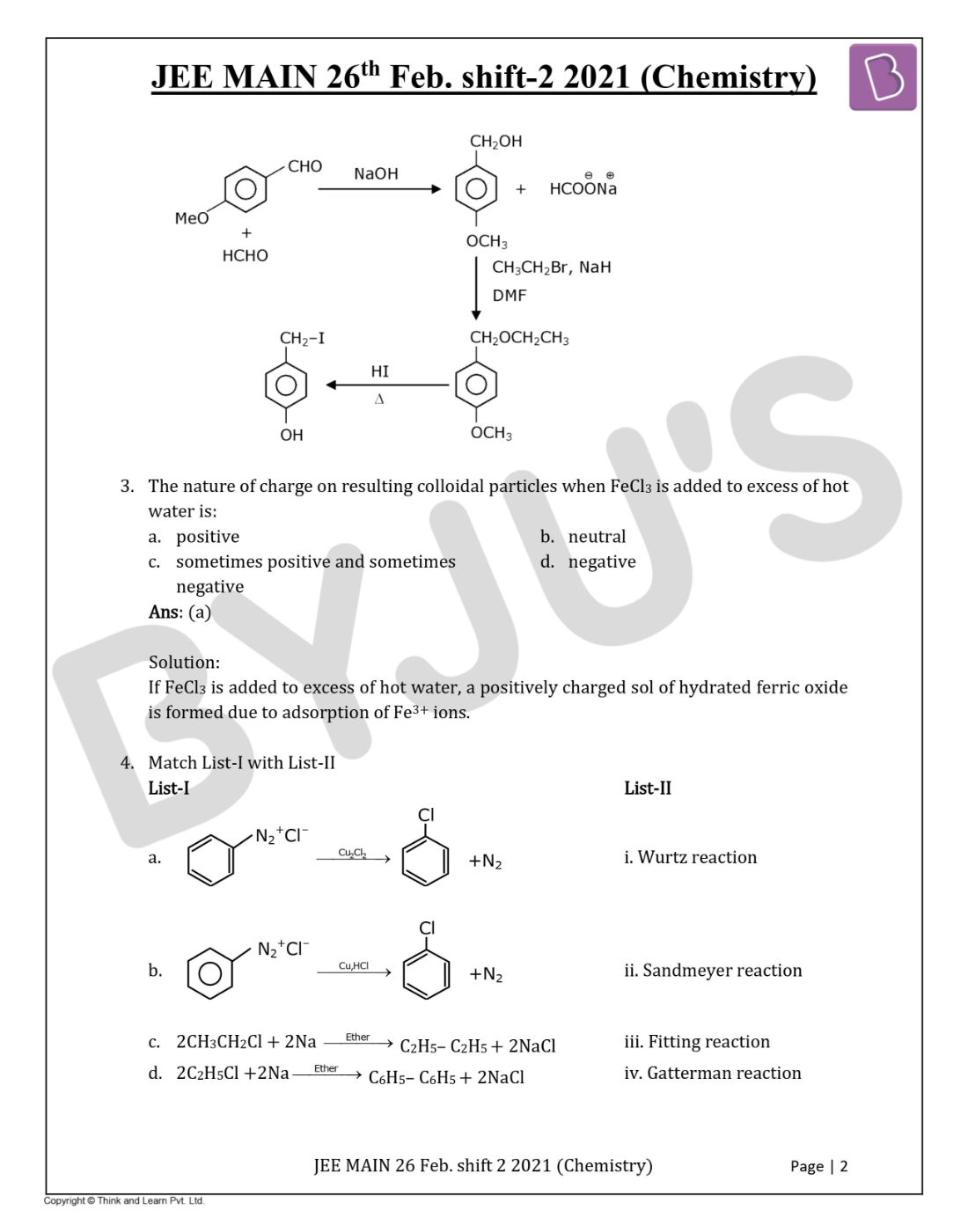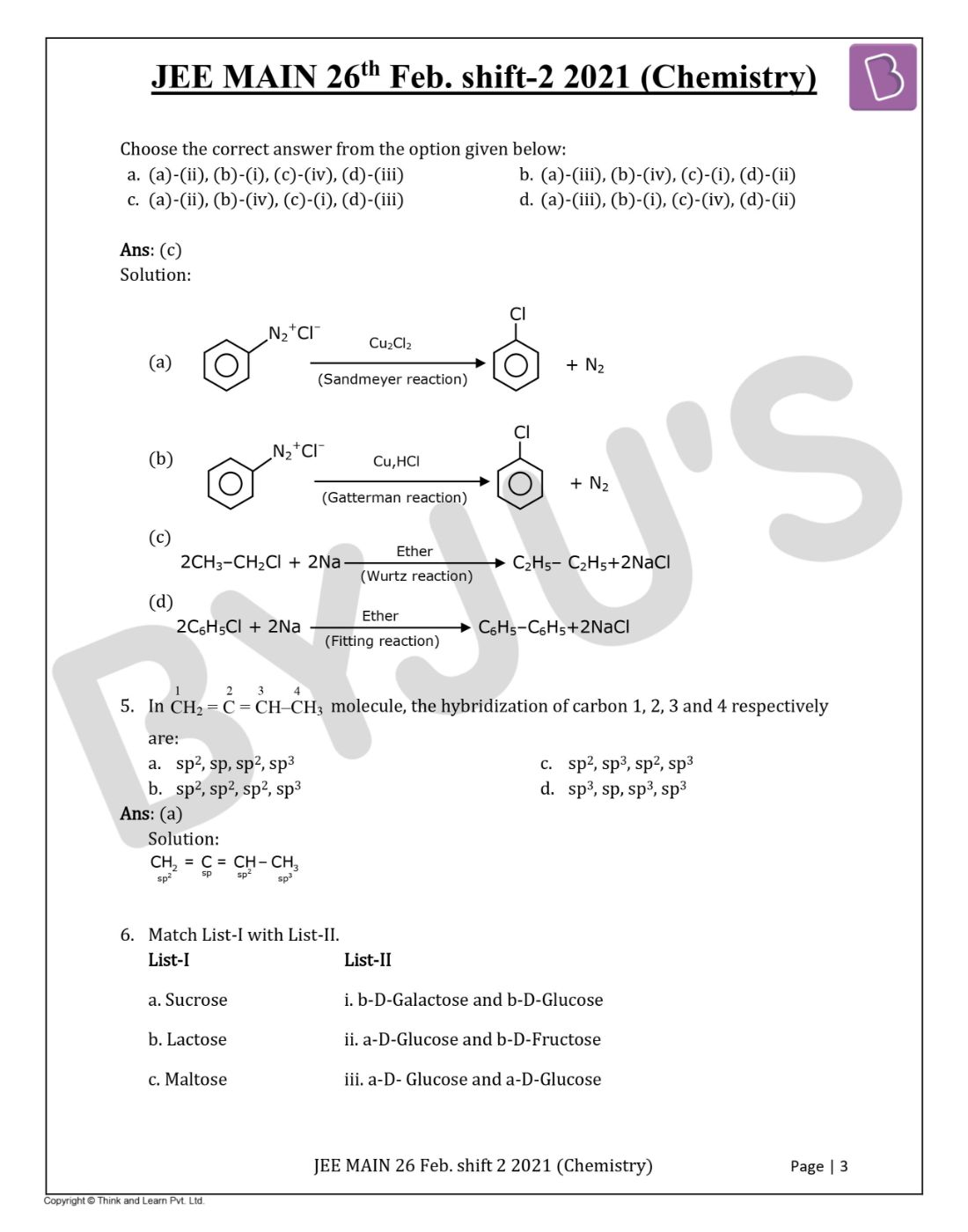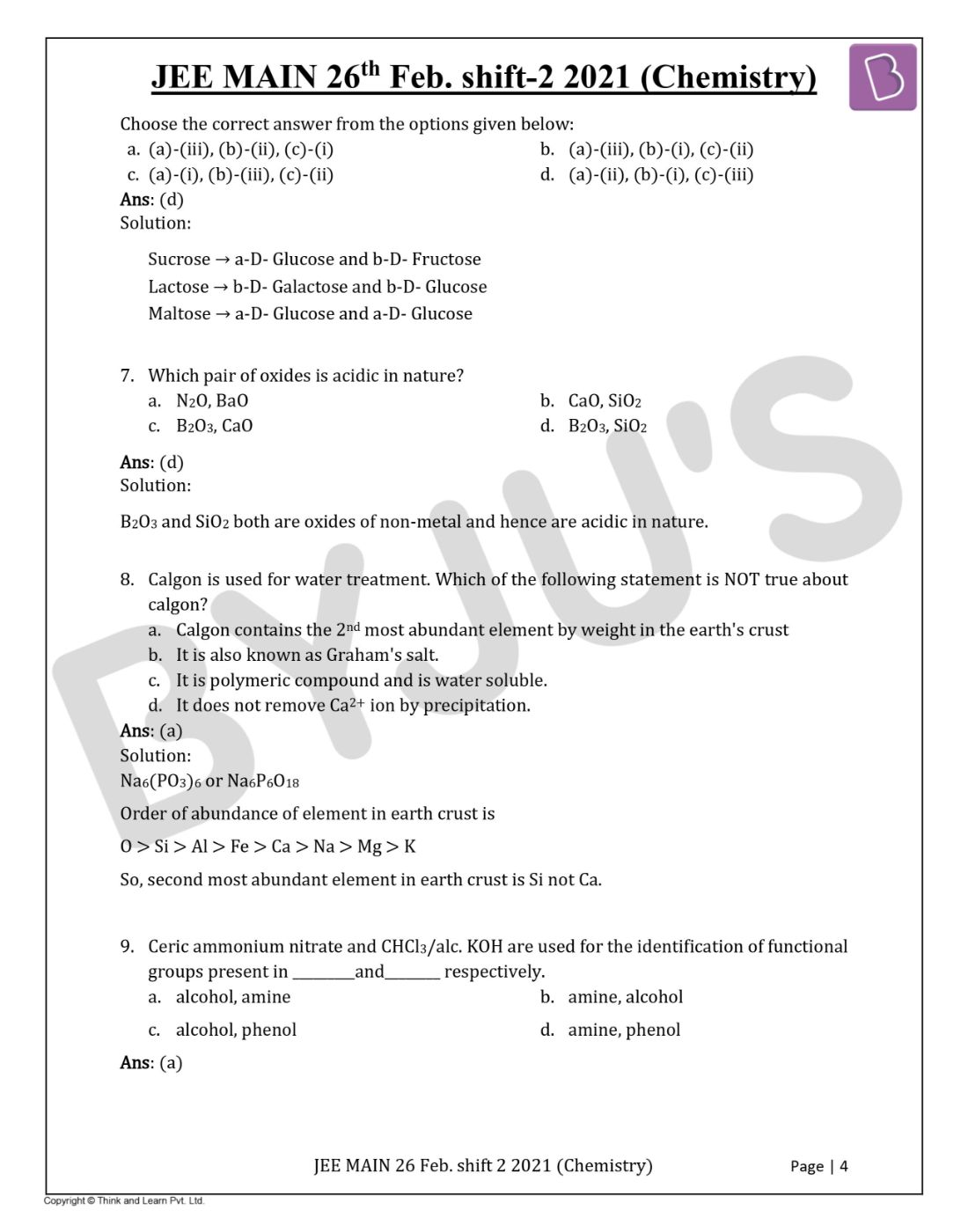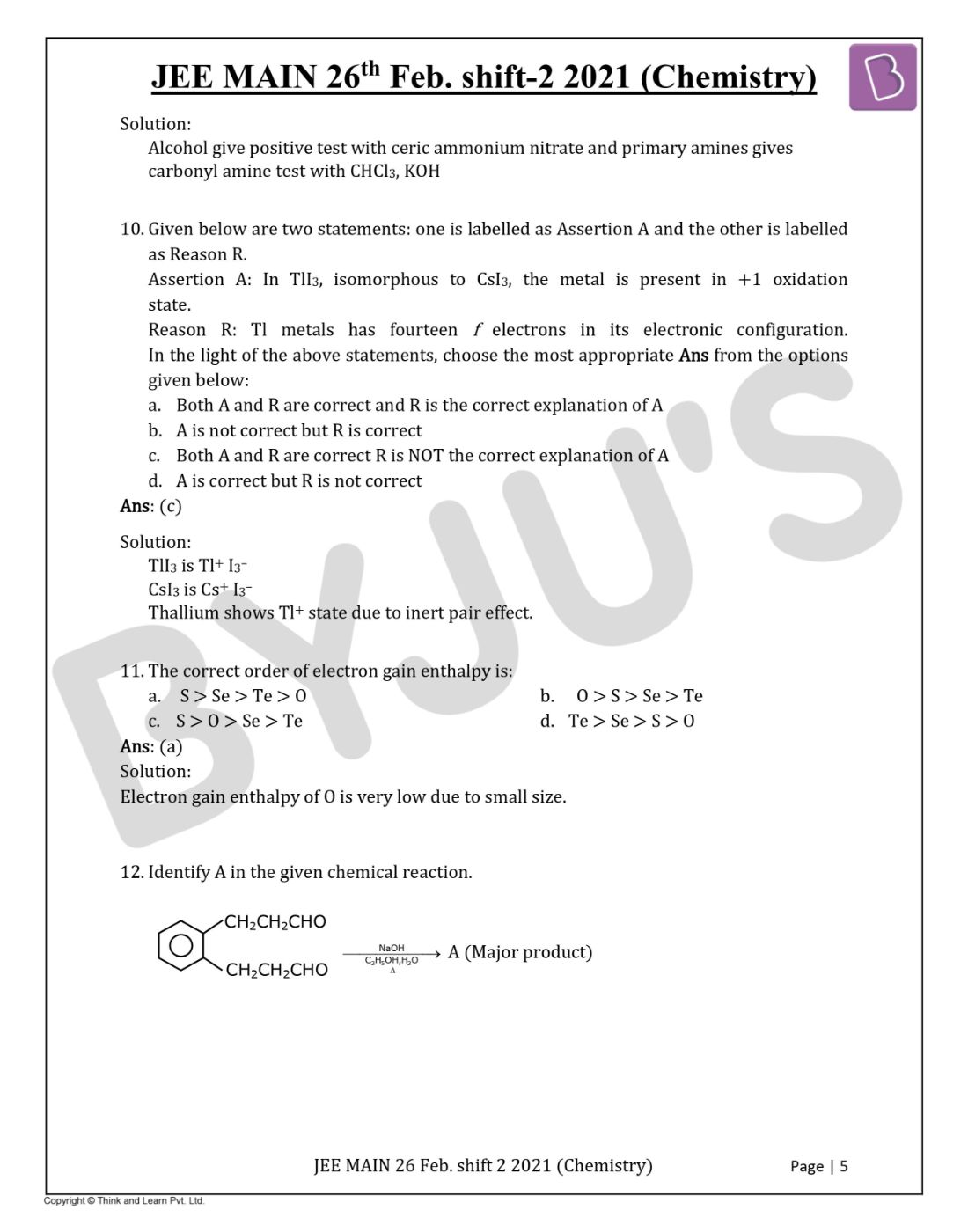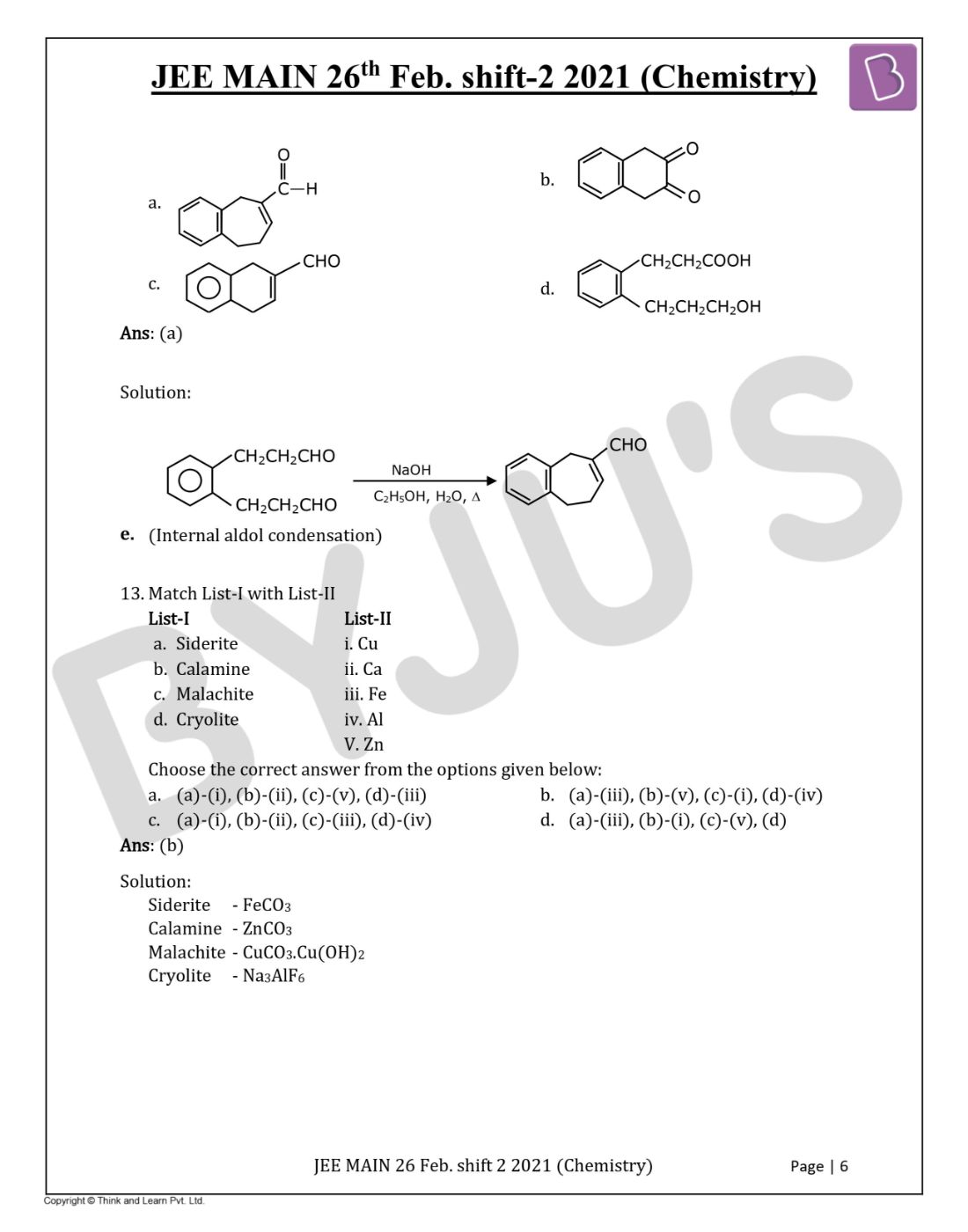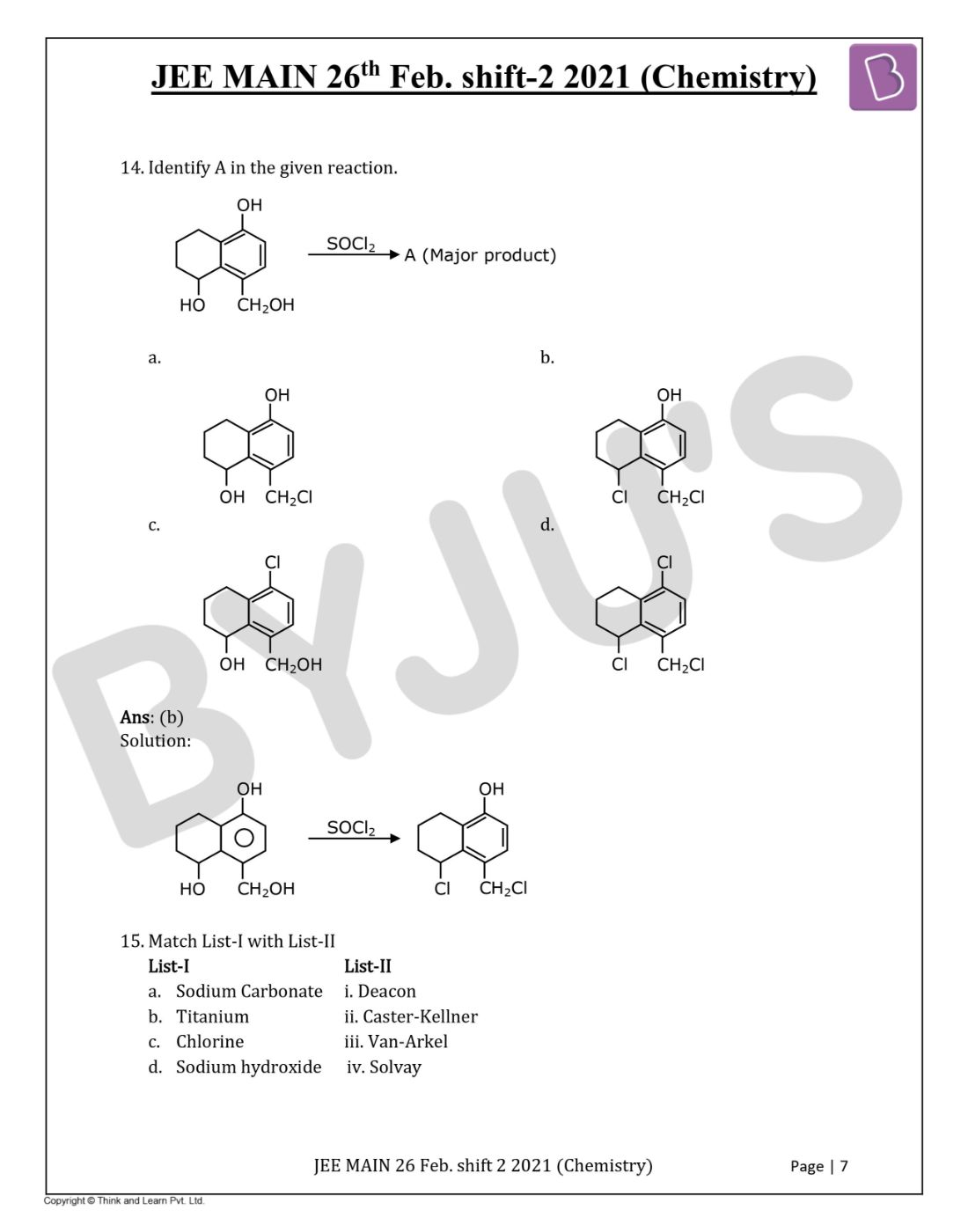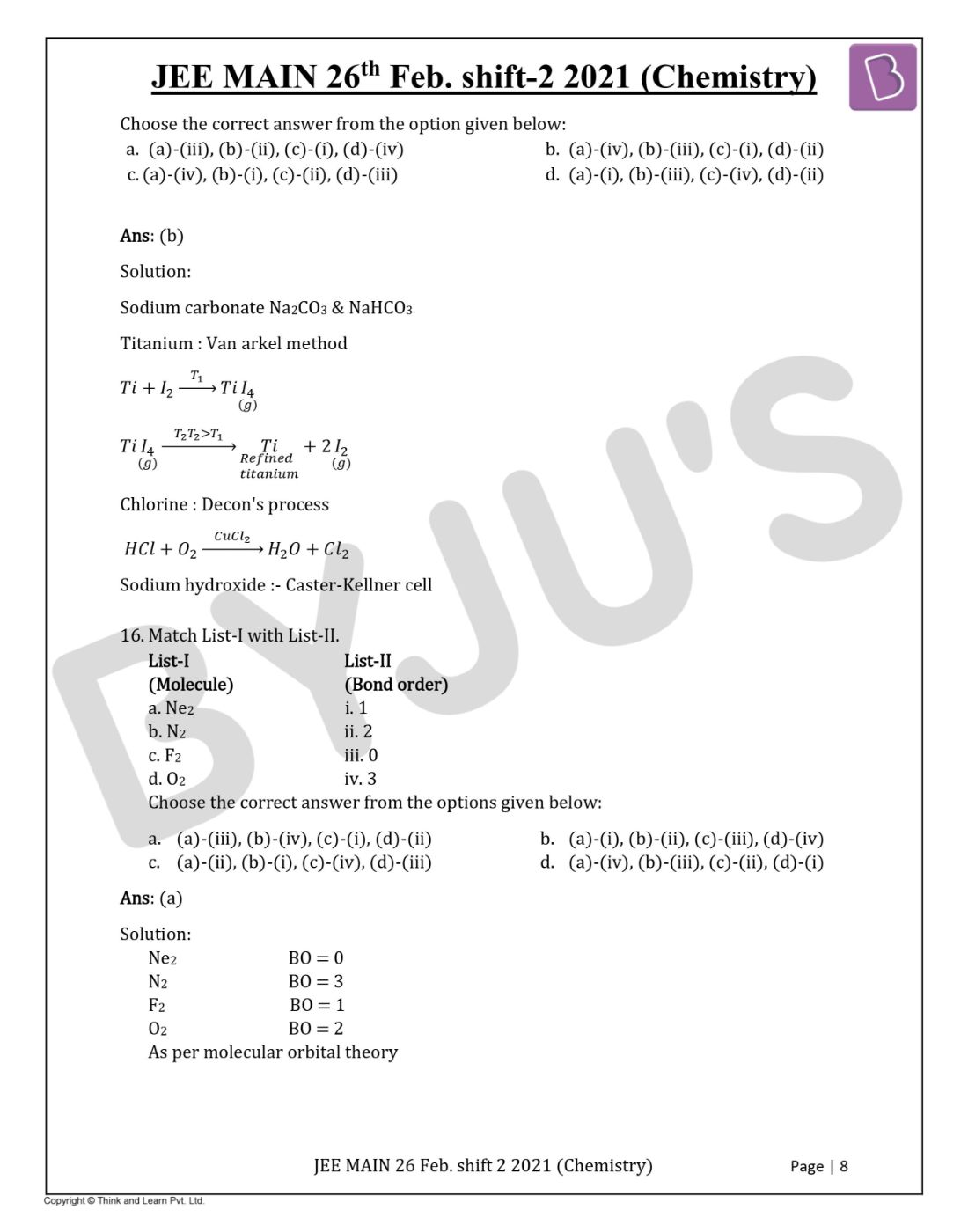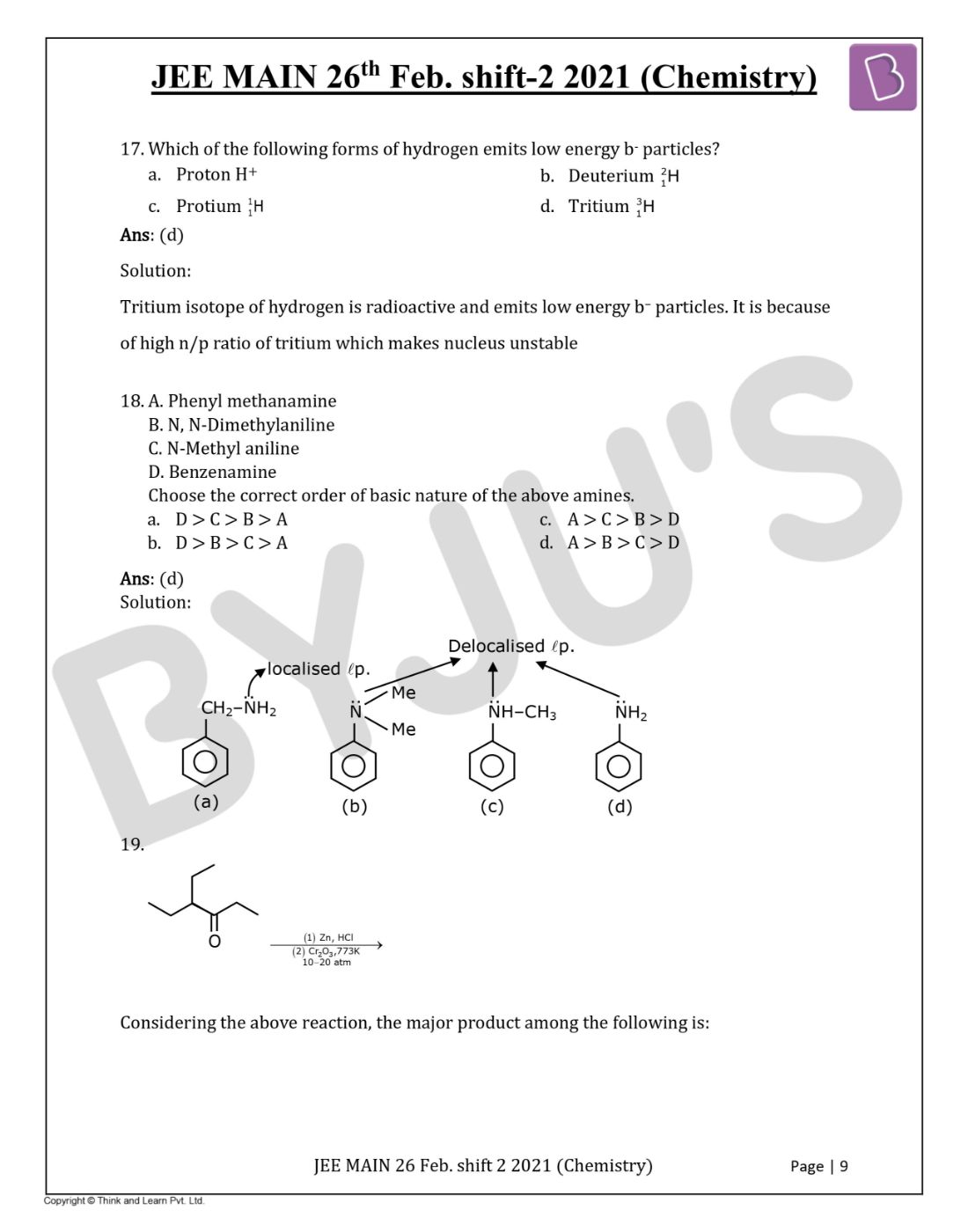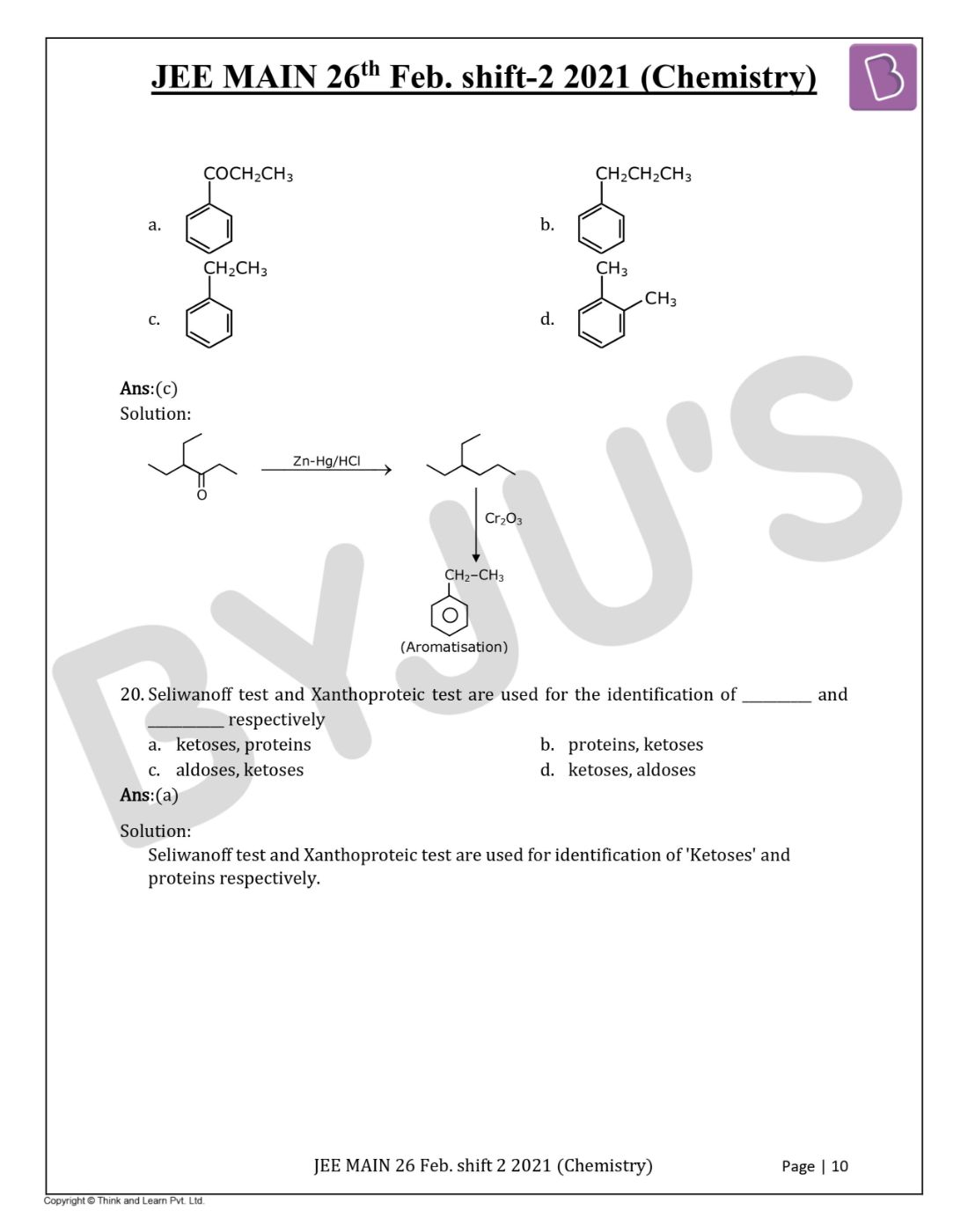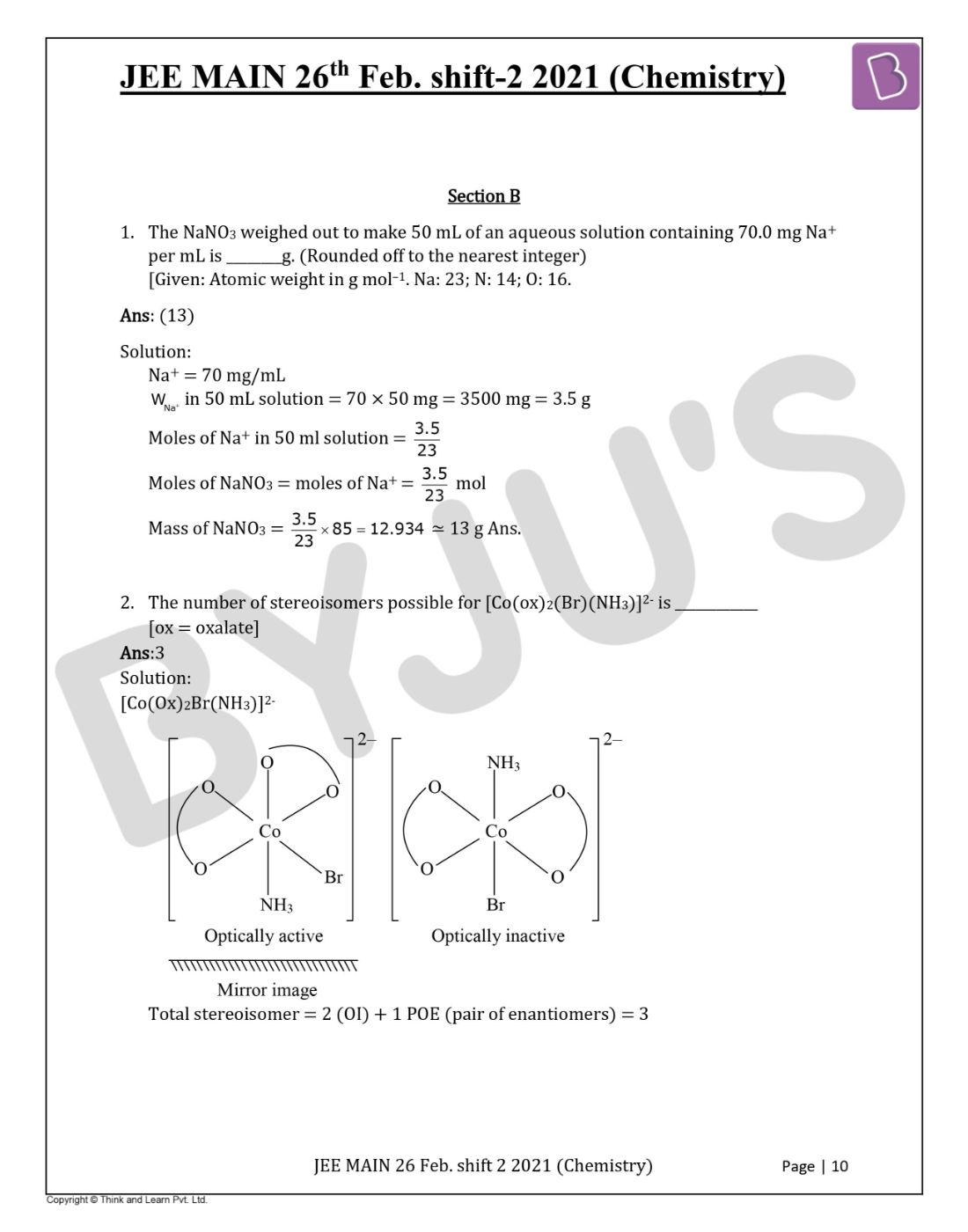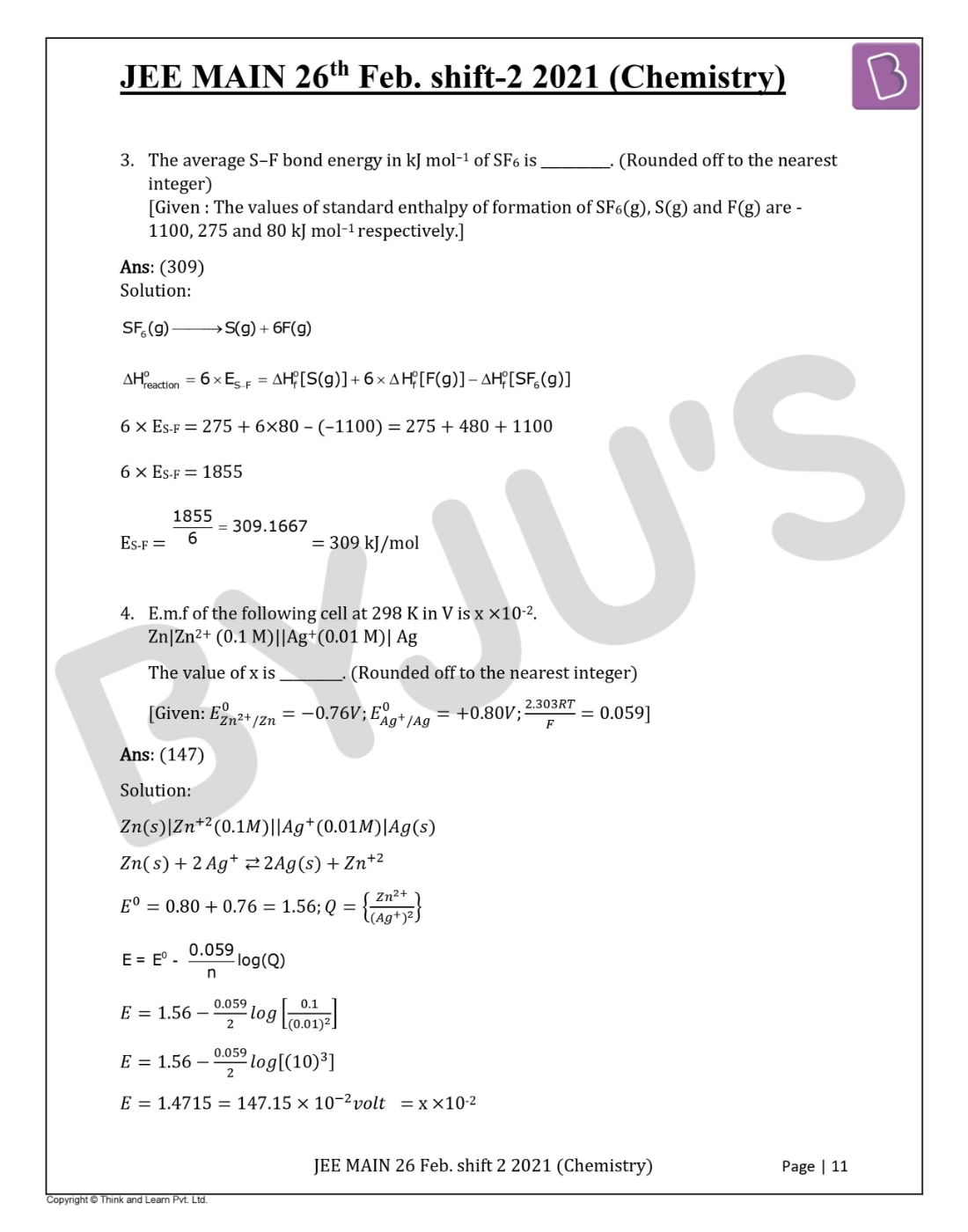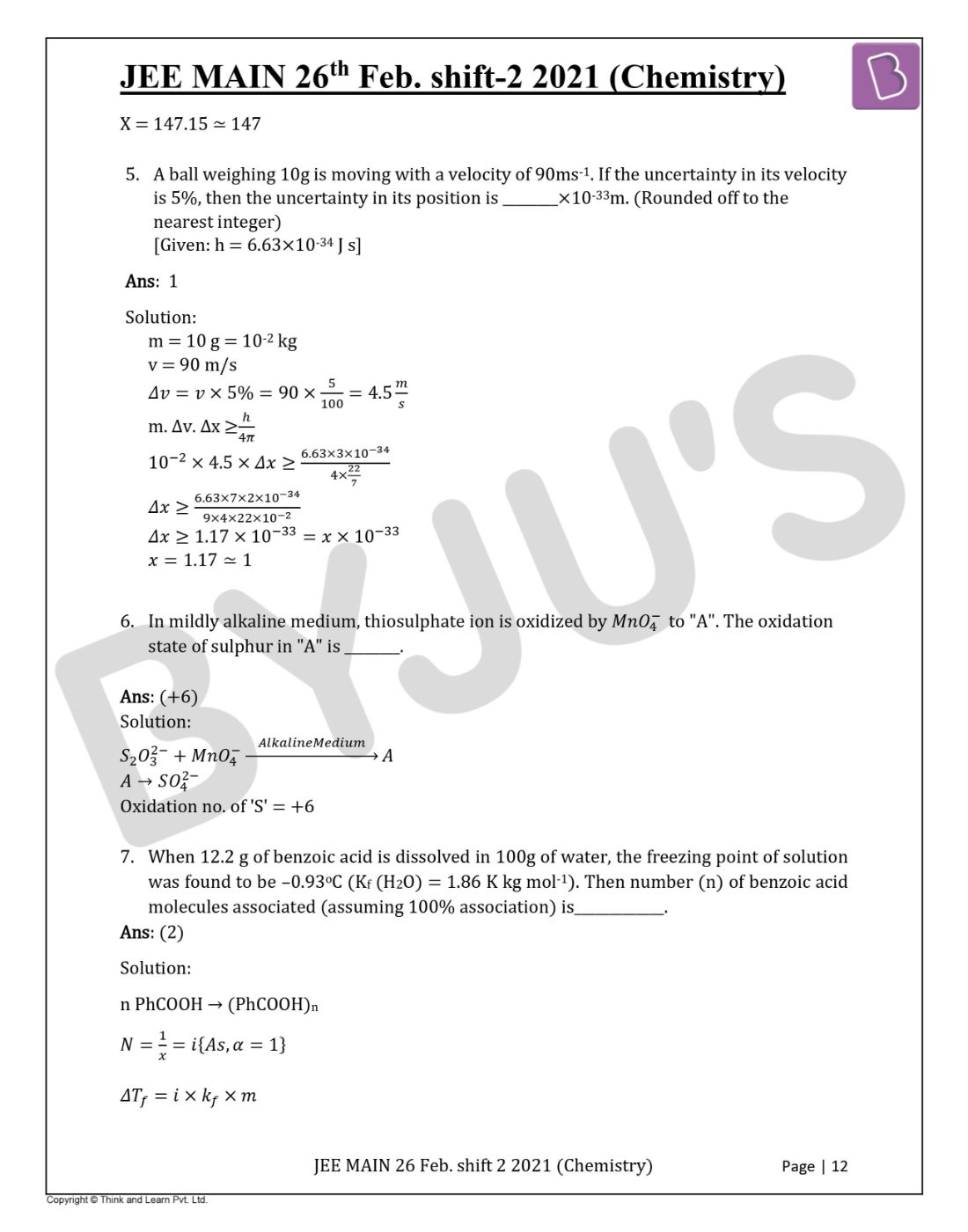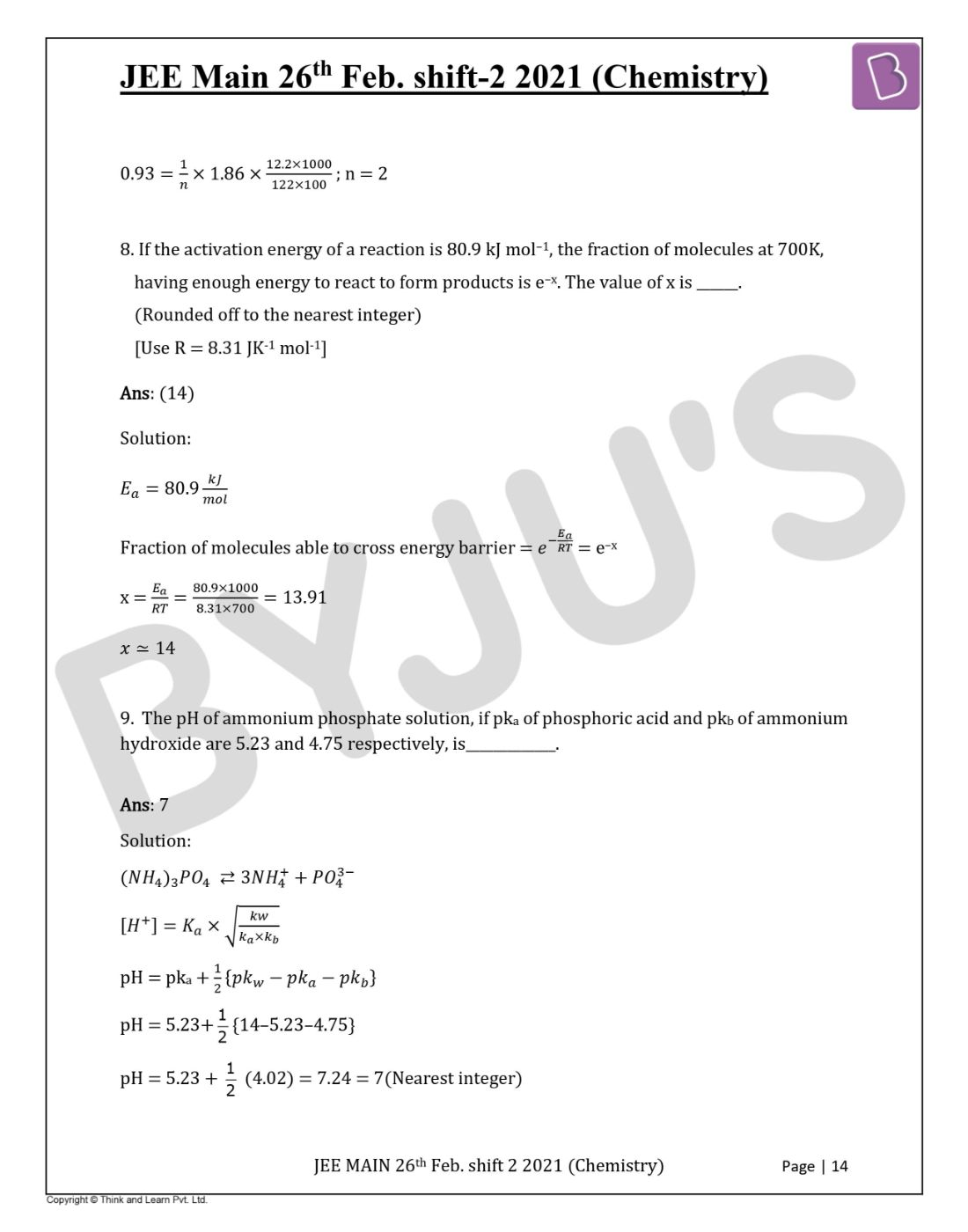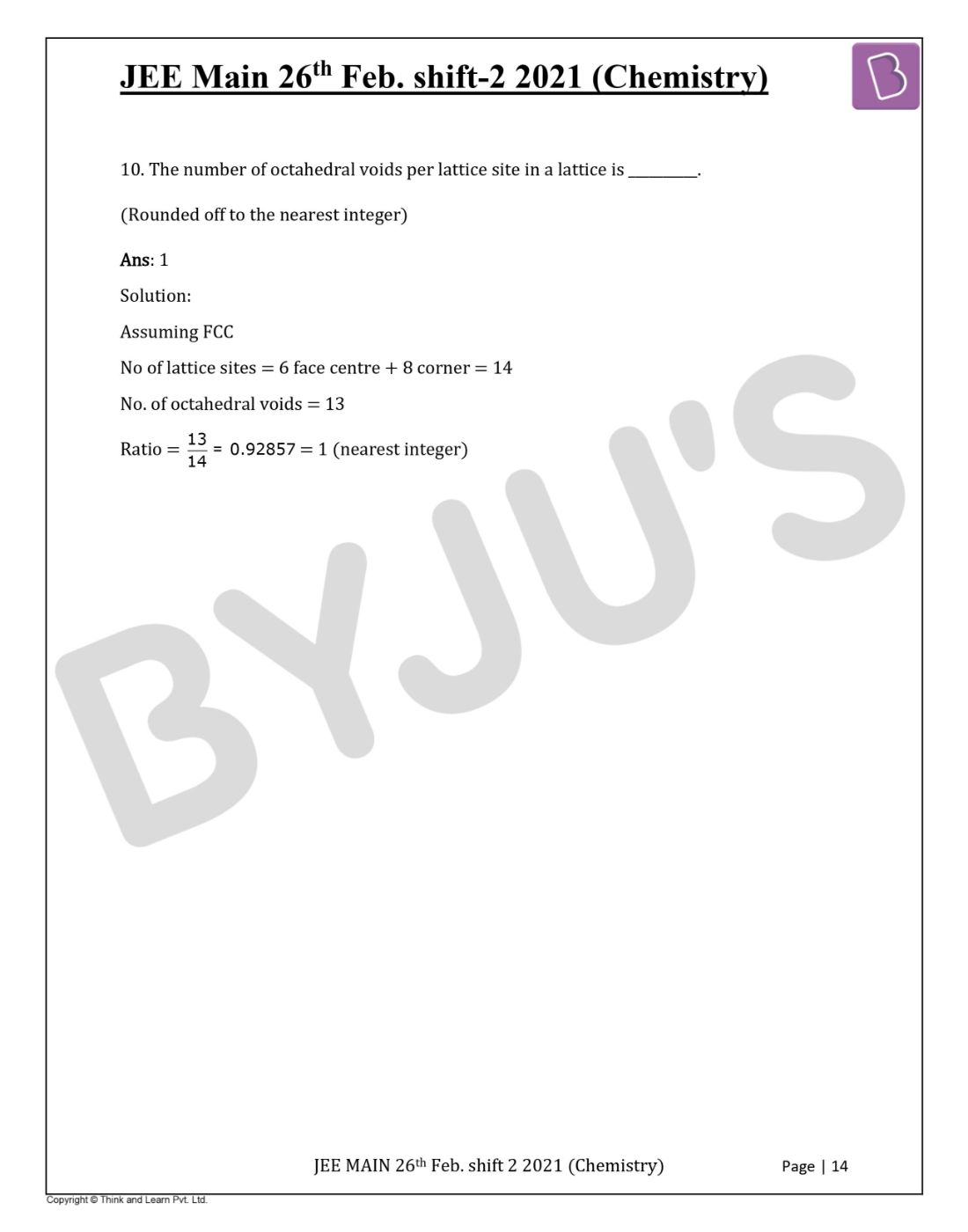Win up to 100% scholarship on Aakash BYJU'S JEE/NEET courses with ABNAT Win up to 100% scholarship on Aakash BYJU'S JEE/NEET courses with ABNAT

# JEE Main 2021 February 26 – Shift 2 Chemistry Question Paper with Solutions

JEE Main 2021 shift 2 Chemistry paper solutions are available here. This study material consists of accurate solutions, prepared by our subject experts. These step by step solutions will help students to understand the problems easily and analyze their performance. Students can have a glance at the question paper and get a better understanding of the question types, difficulty level, marking scheme. Students can either view the paper directly or download it in a PDF format. Detailed solutions are also provided to help the students find the right answer and study effectively for the entrance exam.

JEE Main 2021 26th February Shift 2 Chemistry Question Paper

SECTION A

Question 1. 2,4-DNP test can be used to identify

a. aldehyde
b. halogens
c. ether
d. amineQuestion 2. Identify A in the following chemical reaction.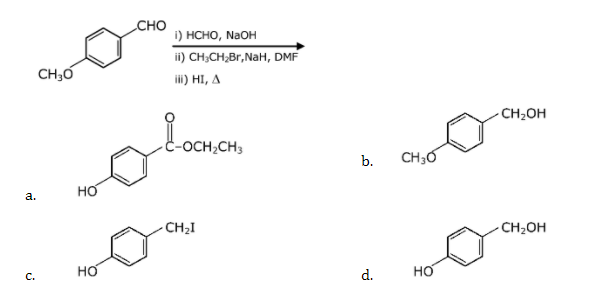Question 3. The nature of charge on resulting colloidal particles when FeCl3 is added to excess of hot water is:

a. positive
b. neutral
c. sometimes positive and sometimes negative
d. negative

If FeCl3 is added to excess hot water, a positively charged sol of hydrated ferric oxide is formed due to the adsorption of Fe3+ ions.

Question 4. Match List-I with List-II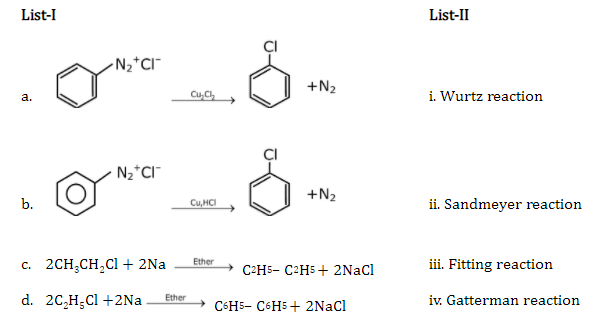Choose the correct answer from the option given below:

a. (a)-(ii), (b)-(i), (c)-(iv), (d)-(iii)
b. (a)-(iii), (b)-(iv), (c)-(i), (d)-(ii)
c. (a)-(ii), (b)-(iv), (c)-(i), (d)-(iii)
d. (a)-(iii), (b)-(i), (c)-(iv), (d)-(ii)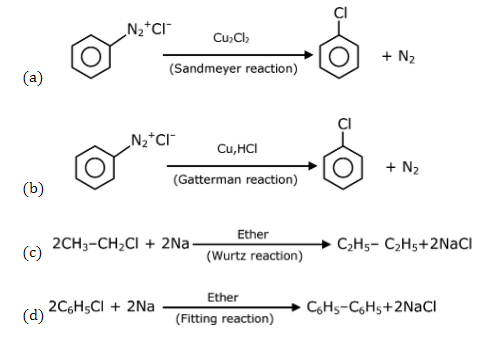Question 5. Inmolecule, the hybridization of carbon 1, 2, 3 and 4 respectively are:

a. sp2, sp, sp2, sp3
b. sp2, sp2, sp2, sp3
c. sp2, sp3, sp2, sp3
d. sp3, sp, sp3, sp3Question 6. Match List-I with List-II.

 List-II List-II a. Sucrose i. b-D-Galactose and b-D-Glucose b. Lactose ii. a-D-Glucose and b-D-Fructose c. Maltose iii. a-D- Glucose and a-D-Glucose

Choose the correct answer from the options given below:

a. (a)-(iii), (b)-(ii), (c)-(i)
b. (a)-(iii), (b)-(i), (c)-(ii)
c. (a)-(i), (b)-(iii), (c)-(ii)
d. (a)-(ii), (b)-(i), (c)-(iii)

Sucrose → a-D- Glucose and b-D- Fructose

Lactose → b-D- Galactose and b-D- Glucose

Maltose → a-D- Glucose and a-D- Glucose

Question 7. Which pair of oxides is acidic in nature?

a. N2O, BaO
b. CaO, SiO2
c. B2O3, CaO
d. B2O3, SiO2

B2O3 and SiO2 both are oxides of non-metal and hence are acidic in nature.

Question 8. Calgon is used for water treatment. Which of the following statements is NOT true about Calgon?

a. Calgon contains the 2nd most abundant element by weight in the earth’s crust
b. It is also known as Graham’s salt.
c. It is a polymeric compound and is water-soluble.
d. It does not remove Ca2+ ion by precipitation.

Na6(PO3)6 or Na6P6O18

Order of abundance of element in earth crust is

O > Si > Al > Fe > Ca > Na > Mg > K

So, the second most abundant element in the earth’s crust is Si not Ca.

Question 9. Ceric ammonium nitrate and CHCl3/alc. KOH are used for the identification of functional groups present in _________and________ respectively.

a. alcohol, amine
b. amine, alcohol
c. alcohol, phenol
d. amine, phenol

Alcohol gives a positive test with ceric ammonium nitrate and primary amines give carbonyl amine test with CHCl3, KOH

Question 10. Given below are two statements: one is labelled as Assertion A and the other is labelled as Reason R.

Assertion A: In TlI3, isomorphous to CsI3, the metal is present in +1 oxidation
state.

Reason R: Tl metals has fourteen f electrons in its electronic configuration.

In the light of the above statements, choose the most appropriate Answer from the options given below:

a. Both A and R are correct and R is the correct explanation of A
b. A is not correct but R is correct
c. Both A and R are correct R is NOT the correct explanation of A
d. A is correct but R is not correct

TlI3 is Tl+ I3

CsI3 is Cs+ I3

Question 11. Thallium shows Tl+ state due to inert pair effect. The correct order of electron gain enthalpy is

a. S > Se > Te > O
b. O > S > Se > Te
c. S > O > Se > Te
d. Te > Se > S > O

Electron gain enthalpy of O is very low due to small size.

Question 12. Identify A in the given chemical reaction.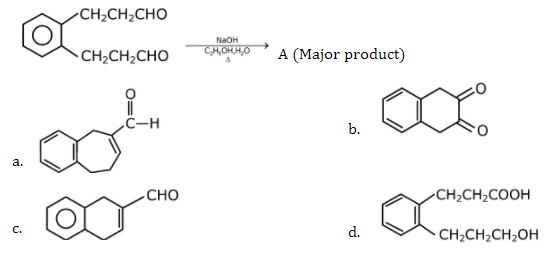a.(Internal aldol condensation)

Question 13. Match List-I with List-II

 List-I List-II a. Siderite i. Cu b. Calamine ii. Ca c. Malachite iii. Fe d. Cryolite iv. Al – v. Zn

Choose the correct answer from the options given below:

a. (a)-(i), (b)-(ii), (c)-(v), (d)-(iii)
b. (a)-(iii), (b)-(v), (c)-(i), (d)-(iv)
c. (a)-(i), (b)-(ii), (c)-(iii), (d)-(iv)
d. (a)-(iii), (b)-(i), (c)-(v), (d)

Siderite – FeCO3

Calamine – ZnCO3

Malachite – CuCO3.Cu(OH)2

Cryolite – Na3AlF6

Question 14. Identify A in the given reaction.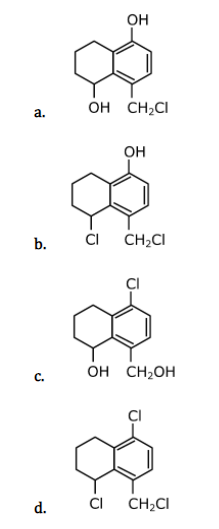Question 15. Match List-I with List-II

 List-I List-II a. Sodium Carbonate i. Deacon b. Titanium ii. Caster-Kellner c. Chlorine iii. Van-Arkel d. Sodium hydroxide iv. Solvay

Choose the correct answer from the option given below

a. (a)-(iii), (b)-(ii), (c)-(i), (d)-(iv)
b. (a)-(iv), (b)-(iii), (c)-(i), (d)-(ii)
c. (a)-(iv), (b)-(i), (c)-(ii), (d)-(iii)
d. (a)-(i), (b)-(iii), (c)-(iv), (d)-(ii)

Sodium carbonate Na2CO3 and NaHCO3

Titanium : Van arkel method

Ti + I2 T1 → TiI4(g)

TiI4(g) T2T2>T1 →TiRefined titanium +2I2(G)

Chlorine: Decon’s process

HCl+O2 CuCl2 → H2O+Cl2

Sodium hydroxide: Caster-Kellner cell

Question 16. Match List-I with List-II.

 List-I List-II (Molecule) (Bond order) a. Ne2 i. 1 b. N2 ii. 2 c. F2 iii. 0 d. O2 iv. 3

Choose the correct answer from the options given below:

a. (a)-(iii), (b)-(iv), (c)-(i), (d)-(ii)
b. (a)-(i), (b)-(ii), (c)-(iii), (d)-(iv)
c. (a)-(ii), (b)-(i), (c)-(iv), (d)-(iii)
d. (a)-(iv), (b)-(iii), (c)-(ii), (d)-(i)

Ne2 BO = 0

N2 BO = 3

F2 BO = 1

O2 BO = 2

As per the molecular orbital theory.

Question 17. Which of the following forms of hydrogen emits low energy b particles?

a. Proton H+
b. Deuterium

$$\begin{array}{l}_{1}^{2}\textrm{H}\end{array}$$

c. Protium
$$\begin{array}{l}_{1}^{1}\textrm{H}\end{array}$$

d. Tritium
$$\begin{array}{l}_{1}^{3}\textrm{H}\end{array}$$

Tritium isotope of hydrogen is radioactive and emits low energy b particles. It is because

of high n/p ratio of the tritium which makes the nucleus unstable.

Question 18. Choose the correct order of the basic nature of the below given amines.

A. Phenyl methanamine

B. N, N-Dimethylaniline

C. N-Methyl aniline

D. Benzenamine

a. D > C > B > A
b. D > B > C > A
c. A > C > B > D
d. A > B > C > DQuestion 19. Considering the given reaction, the major product among the following is: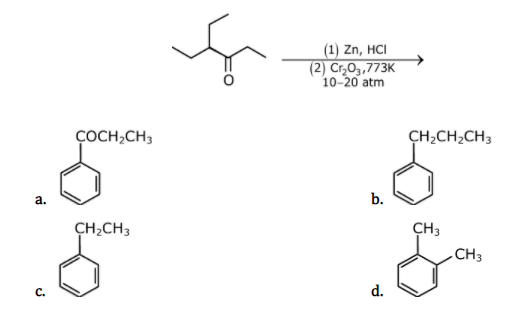Question 20. Seliwanoff test and Xanthoproteic test are used for the identification of __________ and ___________ respectively

a. ketoses, proteins
b. proteins, ketoses
c. aldoses, ketoses
d. ketoses, aldoses

Seliwanoff test and Xanthoproteic test are used for identification of ‘Ketoses’ and proteins respectively.

Section B

Question 1. The NaNO3 weighed out to make 50 mL of an aqueous solution containing 70.0 mg Na+ per mL is ________g. (Rounded off to the nearest integer)
[Given: Atomic weight in g mol–1. Na: 23; N: 14; O: 16.

Na+ = 70 mg/mL

WNa in 50 mL solution = 70 × 50 mg = 3500 mg = 3.5 g

Moles of Na+ in 50 ml solution =3.5/23

Moles of NaNO3 = moles of Na+ = 3.5/23 mol

Mass of NaNO3 =3.5 / 23 × 85 = 12.94 13 g Ans.

Question 2. The number of stereoisomers possible for [Co(ox)2(Br)(NH3)]2- is ____________. [ox = oxalate]

[Co(Ox)2Br(NH3)]2-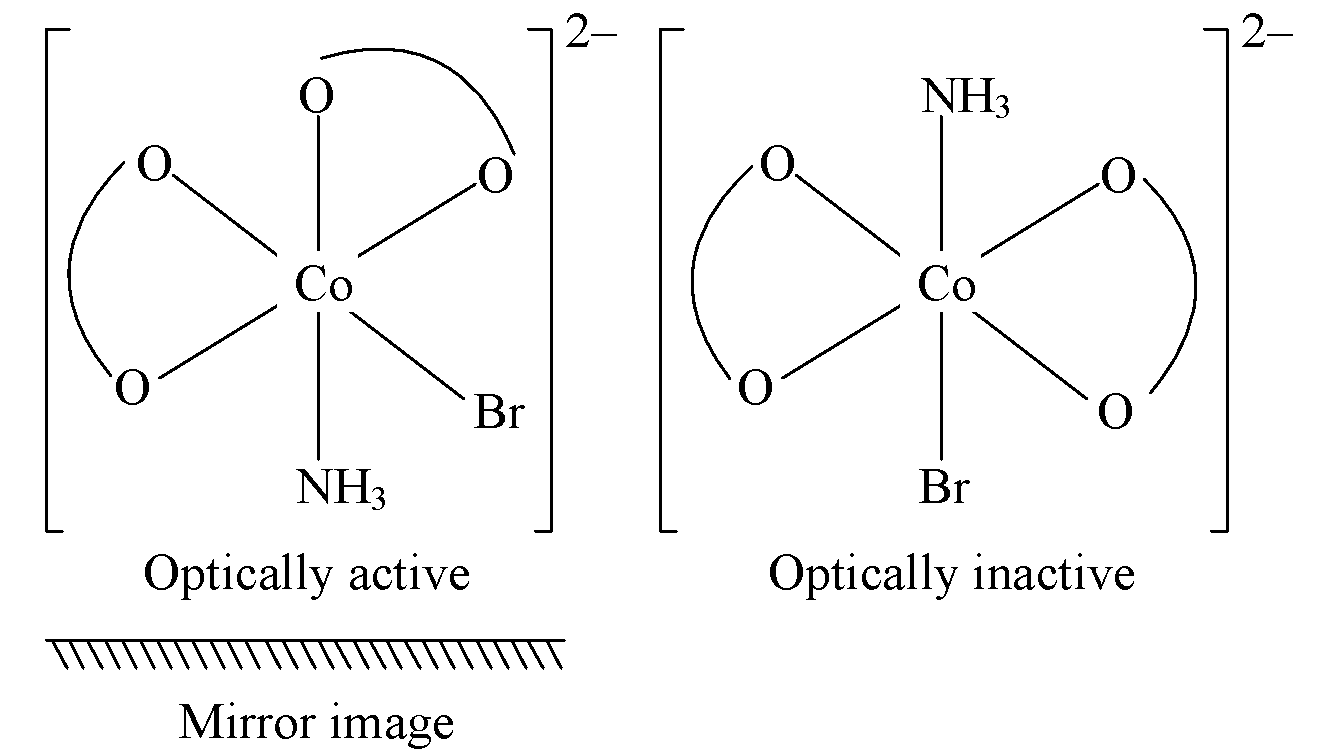Total stereoisomer = 2 (OI) + 1 POE (pair of enantiomers) = 3

Question 3. The average S–F bond energy in kJ mol–1 of SF6 is __________. (Rounded off to the nearest integer)
[Given : The values of standard enthalpy of formation of SF6(g), S(g) and F(g) are – 1100, 275 and 80 kJ mol–1 respectively.]

SF6(g) → S(g) + 6F(g)6 × ES-F = 275 + 6×80 – (–1100) = 275 + 480 + 1100

6 × ES-F = 1855

ES-F = 1855/6 = 309.1667 = 309 kJ/mol

Question 4. E.m.f of the following cell at 298 K in V is x ×10-2.

Zn|Zn2+ (0.1 M)||Ag+(0.01 M)| Ag

The value of x is _________. (Rounded off to the nearest integer)

$$\begin{array}{l}[Given: E_{Zn^{2}/Zn}^{0} = -0.76; E_{Ag^{+}/Ag}^{0} = +0.80V; \frac{2.303RT}{F} = 0.059]\end{array}$$

Zn(s)|Zn+2(0.1M)||Ag+(0.01M)|Ag(s)

( ) + 2 ? 2Ag(s) + Zn+2

Eo = 0.80 + 0.76 = 1.56; Q = {Zn2+ / (Ag2)2}

E = Eo – 0.059/n log(Q)

E = 1.56 – 0.059 / 2 log [0.1 / (0.01)2]

E = 1.56 – 0.059 / 2 log [(10)3]

E = 1.4715 = 147.15 × 10-2 volt = x × 10-2

X = 147.15 ? 147

Question 5. A ball weighing 10g is moving with a velocity of 90ms-1. If the uncertainty in its velocity is 5%, then the uncertainty in its position is ________×10-33m. (Rounded off to the nearest integer)

[Given: h = 6.63×10-34 J s]

m = 10 g = 10-2 kg

v = 90 m/s

Δv = v × 5% = 90 × 5 / 100 = 4.5 m/sΔx ≥ 1.17 × 10-33 = x × 10-33

x = 1.17?1

Question 6. In a mildly alkaline medium, thiosulphate ion is oxidized by to “A”. The oxidation state of sulphur in “A” is ________.

S2O2-3 + MnO4  Alkaline Medium →A

A → SO2-4

Oxidation no. of ‘S’ = +6

Question 7. When 12.2 g of benzoic acid is dissolved in 100g of water, the freezing point of solution was found to be –0.93oC (Kf (H2O) = 1.86 K kg mol-1). Then the number (n) of benzoic acid molecules associated (assuming 100% association) is_____________.

n PhCOOH (PhCOOH)n

N = 1/x = i{As, α=1}

?Tf = i × kf × m

0.93 = 1/n × 1.86 × 12.2 × 1000 / 122 × 100; n = 2

Question 8. If the activation energy of a reaction is 80.9 kJ mol–1, the fraction of molecules at 700K, having enough energy to react to form products is e–x. The value of x is ______. (Rounded off to the nearest integer)

[Use R = 8.31 JK-1 mol-1]

Ea = 80.9 kJ / mol

Fraction of molecules able to cross energy barrier = e – Ea / RT = e-x

x = Ea / RT = 80.9 × 1000 / 8.31 × 700 = 13.91

x ? 14

Question 9. The pH of ammonium phosphate solution, if pka of phosphoric acid and pkb of ammonium hydroxide are 5.23 and 4.75 respectively, is_____________.

$$\begin{array}{l}\left ( NH_{4} \right )_{3}PO_{4}\leftrightharpoons 3NH_{4}^{+} + PO_{4}^{3-}\end{array}$$
$$\begin{array}{l}[H^{+}] = K_{a}\times \sqrt{\frac{kw}{k_{a}\times k_{b}}}\end{array}$$

pH = pka + ½ {pkw – pka – pkb}

pH = 5.23 + ½ {14–5.23–4.75}

pH = 5.23 + ½ (4.02) = 7.24 = 7(Nearest integer)

Question 10. The number of octahedral voids per lattice site in a lattice is __________.

(Rounded off to the nearest integer)

Assuming FCC

No of lattice sites = 6 face centre + 8 corner = 14

No. of octahedral voids = 13

Ratio = 13/14 = 0.92857 = 1 (nearest integer)

#### Video Solutions- February 2021 Question Papers